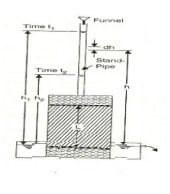Home | | Soil Mechanics | Soil Water: Permeability

# Soil Water: PermeabilityPermeability is defined as the property of a porous material which permits the passage or seepage of water (or other fluids) through its interconnecting voids.

PERMEABILITY:

Permeability is defined as the property of a porous material which permits the passage or seepage of water (or other fluids) through its interconnecting voids. A material having continuous voids is called permeable. Gravels are highly permeable while stiff clay is the least permeable and hence such clay may be termed impermeable for all practical purposes.

The flow of water through soils may either be a laminar flow or a turbulent flow. In laminar flow, each fluid particle travels along a definite path which never crosses the path of any other particle. In turbulent flow, the paths are irregular and twisting, crossing and recrossing at random (Taylor, 1948).

In most of the practical flow problems in soil mechanics, the flow is laminar. The study of seepage of water through soil is important for the following engineering problems :

1. Determination of rate of settlement of a saturated compressible soil layer.

2. Calculation of seepage through the body of earth dams, and stability of slopes.

3. Calculation of uplift pressure under hydraulic structures and their safety against piping.

4. Ground water flow towards wells and drainage of soil.

1 Coefficient of permeability (or) permeability.

It is defined as the average velocity of flow that will occur through the total cross-sectional are of soil under unit hydraulic gradient. The coefficient of permeability is denoted as K. It is usually expressed as cm/sec (or) m/day (or) feet/day.

Darcy's Law:

When the flow is laminar in a saturated soil, the rate of flow or discharge per unit time is proportional to the hydraulic gradient.

Q=kia

q = discharge per unit time

v=q/A=ki

A = total cross-sectional area of soil mass, perpendicular to the direction of flow

k = Darcy's coefficient of permeability

v = velocity of flow, or average discharge velocity.If a soil sample of length L and cross- sectional area A, is subjected to differential head of water, the hydraulic gradient i will be equal to

q=h1-h2 / L  (A)2 Factors affecting permeability:

1. Grain size

2. Properties of the pore fluid

3. Voids ratio of the soil

4. Structural arrangement of the soil particles

5. Entrapped air and foreign-matter

6. Adsorbed water in clayey soils.

3 The coefficient of permeability can be determined by the following methods:

(a) Laboratory methods

(b) Field methods

(1)Pumping-out tests.

(2)Pumping-in tests.

(c) Indirect methods

(1)Computation from grain size or specific surface.

(2)Horizontal capillarity test

(3)Consolidation test data.

The co efficient of permeability of a soil sample determined by the constant water pressure. The test is conducted with a fixed water level. Permeability is measured in cm/sec.Co-fficient permeability by constant head method

K=QL/Ah

Constant head permeability most used for coarse grained soils.

A Stand pipe of known cross sectional are is fitted over the permeameter and water is allows to run down. The water level in the stand pipe constantly falls as water flows.

The head of water on the stand pipe at time intervals is observed and the data used to determine the co efficient of permeability.

K=(2.303)qL/At . log10(h1/h2)Falling head permeability test relatively used for less permeable soils.

Study Material, Lecturing Notes, Assignment, Reference, Wiki description explanation, brief detail
Civil : Soil Mechanics -Soil Water And Water Flow : Soil Water: Permeability |